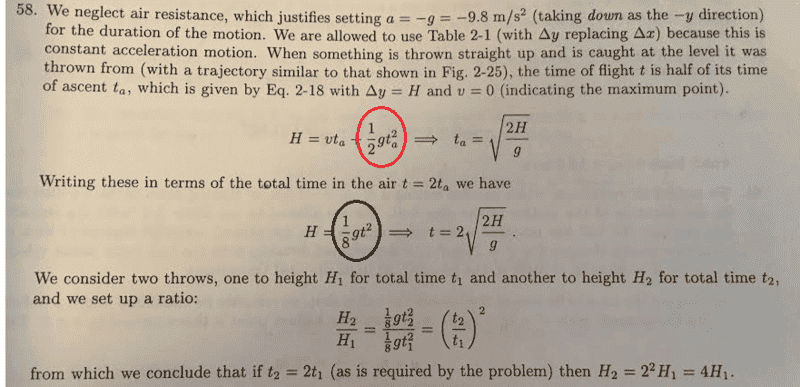# Doubling the time spent in the air by a thrown object

marksyncm

## Homework Statement

An object is thrown straight up from the ground and reaches a maximum height of H. How high up do we need to throw the object to double the time it spends in the air? Assume no air friction.

## Homework Equations

##\Delta x = vt+\frac{g}{2}t^2##

## The Attempt at a Solution

I solved this as follows:

1) Setting ##v=0## in the equation above, the time it takes the object to reach maximum height is ##t=\sqrt{\frac{2H}{g}}##
2) Total time spent in the air is equal to double the above, so to double the time spent in the air I just need to double the time ##t## it takes to reach peak height: ##2t = 2\sqrt{\frac{2H}{g}} = \sqrt{\frac{8H}{g}}##
3) Therefore, we quadrupled the height to double the time spent in the air.

What I do not understand is the solution presented to this problem in my textbook (Resnick/Halliday). Here's a screenshot:I do not understand how we can go straight from the part encircled in red to the part encircled in black. I can see how ##H=\frac{1}{8}gt^2## follows from ##t=2\sqrt{\frac{2H}{g}}##, but I do not see how it follows from ##H=vt_a+\frac{1}{2}gt_a^2## simply based on the fact that the time is being doubled. Is there a step missing, or am I missing something?

## Answers and Replies

Homework Helper
Gold Member
Compare the right hand side of both of those two equations.

Rearrange the right hand side of the second to get the left hand side.

•marksyncm
marksyncm
Ahhh, I see it. Thanks.

Mentor
Since ##t## is double ##t_a##, if the form ##\frac{1}{2} g t^2## is to give the same value H, then ##t## must be divided by two. That is,

##H = \frac{1}{2} g t_a^2 = \frac{1}{2} g \left( \frac{t}{2} \right)^2##

•marksyncm